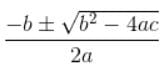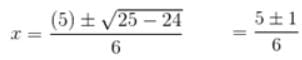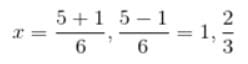Test Description

## 20 Questions MCQ Test Additional Documents & Tests for Class 10 | Test: Quadratic Equations (Hard)

Test: Quadratic Equations (Hard) for Class 10 2022 is part of Additional Documents & Tests for Class 10 preparation. The Test: Quadratic Equations (Hard) questions and answers have been prepared according to the Class 10 exam syllabus.The Test: Quadratic Equations (Hard) MCQs are made for Class 10 2022 Exam. Find important definitions, questions, notes, meanings, examples, exercises, MCQs and online tests for Test: Quadratic Equations (Hard) below.
 1 Crore+ students have signed up on EduRev. Have you?
Test: Quadratic Equations (Hard) - Question 1

### The values of k for which the roots are real and equal of the following equation kx2 —2√5x + 4 = 0 is k = 5/4

Detailed Solution for Test: Quadratic Equations (Hard) - Question 1 => Given a quadratic equation is kx2 - 2-√5x + 4 = 0, comparing it with ax2 + bx + c = 0.
Test: Quadratic Equations (Hard) - Question 2

### What is the degree of a quadratic equation?

Detailed Solution for Test: Quadratic Equations (Hard) - Question 2 The standard form of quadratic equation is ax2 + bx + c = 0, a ≠ 0. So the degree of a quadratic equation is 2
Test: Quadratic Equations (Hard) - Question 3

### Which of the following is not quadratic equation?

Detailed Solution for Test: Quadratic Equations (Hard) - Question 3 (a) (x − 2)2 + 1 = (2x – 3)

x2 – 4x + 4 + 1 = 2x – 3

x2 – 4x + 4 + 1 – 2x + 3 = 0

x2 – 6x + 8 = 0

(b) (x + 2)3 = x3 – 4

x3 + 6x2 + 12x + 8 = x3 – 4

6x2 + 12x + 12 = 0

(c) x(2x + 3) = x2 + 1

2x2 + 3x = x2 + 1

x2 + 3x - 1 = 0

(d) x(x + 1) + 8 = (x + 2) (x – 2)

x2 + x + 8 = x2 – 4

x + 12 = 0

This is not a Quadratic equation.

Test: Quadratic Equations (Hard) - Question 4

If the sum of the roots of a quadratic equation is 5 and the product of the roots is also 5, then the equation is

Detailed Solution for Test: Quadratic Equations (Hard) - Question 4 For a quadratic equation ax2 + bx + c = 0,

sum of roots = −ba

product of roots = ca.

sum of roots = 5 = −ba

product of roots = 5 = ca,

Thus, quadratic equation is x2 − 5x + 5 = 0

Test: Quadratic Equations (Hard) - Question 5

A rectangular field has an area of 3 sq. units. The length is one more than twice the breadth ‘x’. Frame an equation to represent this.

Detailed Solution for Test: Quadratic Equations (Hard) - Question 5 Area of rectangle = length × breadth

Given, length = (2 × breadth + 1)

Let the breadth of the field be x.

Length of the field = 2x + 1

Area of the rectangular field = x (2x + 1) =3

2x2 + x = 3

2x2 + x − 3 = 0

Test: Quadratic Equations (Hard) - Question 6

If a train travelled 5 km/hr faster, it would take one hour less to travel 210 km. The speed of the train is :

Detailed Solution for Test: Quadratic Equations (Hard) - Question 6 Let the speed of the train be x km/hr.

Distance travelled = 210 km

Time taken to travel 210 km = 210 / x hours

When the speed is increased by 5 km/h, the new speed is (x + 5)

Time taken to travel 210 km with the new speed is 210 / (x + 5) hours

According to the question,

210 / x − 210 / (x + 5) = 1

⇒ 210(x + 5) − 210x = x(x + 5)

⇒ 210x + 1050 − 210x = x2 + 5x

⇒ x2 + 5x − 1050 = 0

⇒ (x + 35)(x − 30) = 0

⇒ x = −35, 30

The speed cannot be negative.

Thus, the speed of the train is 30 km/hr.

Test: Quadratic Equations (Hard) - Question 7

If the solutions of the equation x2 + 3x − 18 = 0 are -6, 3 then the roots of the equation 2(x2 + 3x − 18) = 0 are

Detailed Solution for Test: Quadratic Equations (Hard) - Question 7 The roots of a quadratic equation do not change when it is multiplied by a constant non-zero real number. So when the equation x2 + 3x − 18 = 0 is multiplied by 2, the roots still remain the same i.e. -6, 3.
Test: Quadratic Equations (Hard) - Question 8

The square of (5x + 1) is equal to 16. What isx?

Detailed Solution for Test: Quadratic Equations (Hard) - Question 8 Converting statement into an equation-

⇒ (5x + 1)2 = 16 (Applying (a + b)2 formula)

⇒ 5x + 1 = ± 4(Taking square root on both sides)

⇒ 5x = -5, 3

⇒ x = −1, 3 / 5

Test: Quadratic Equations (Hard) - Question 9

Find the roots of the quadratic equation: x2 + 2x - 15 = 0?

Detailed Solution for Test: Quadratic Equations (Hard) - Question 9 x2 + 5x - 3x - 15 = 0

x(x + 5) - 3(x + 5) = 0

(x - 3)(x + 5) = 0

=> x = 3 or x = -5.

Test: Quadratic Equations (Hard) - Question 10

Using the method of completion of squares find one of the roots of the equation 2x2 − 7x + 3 = 0. Also, find the equation obtained after completion of the square.

Detailed Solution for Test: Quadratic Equations (Hard) - Question 10 2x2 − 7x + 3 = 0

Dividing by the coefficient of x2, we get

x2 − 7 / 2x + 3 / 2 = 0; a = 1, b = 7 / 2, c = 3 / 2

Adding and subtracting the square of b / 2 = 7 / 4, (half of coefficient of x)

We get, [x2 − 2 (7 / 4) x + (7 / 4)2] − (7 / 4)2 + 3 / 2 = 0

The equation after completing the square is: (x − 7 / 4)2 − 25 / 16 = 0

Taking square root, (x − 7 / 4) = (±5 / 4)

Taking positive sign 5 / 4, x = 3

Taking negative sign −5 / 4, x = 1 / 2

Test: Quadratic Equations (Hard) - Question 11

If the roots of a quadratic equation are 20 and -7, then find the equation?

Detailed Solution for Test: Quadratic Equations (Hard) - Question 11 Any quadratic equation is of the form

x2 - (sum of the roots)x + (product of the roots) = 0 ---- (1)

where x is a real variable. As sum of the roots is 13 and product of the roots is -140, the quadratic equation with roots as 20 and -7 is: x2 - 13x - 140 = 0.

Test: Quadratic Equations (Hard) - Question 12

Find the roots of the equation 5x2–6x–2=0 by the method of completing the square.

Detailed Solution for Test: Quadratic Equations (Hard) - Question 12 Multiplying the equation throughout by 5, we get 25x2 – 30x – 10 = 0

This is the same as:

(5x)2 – [2× (5x) ×3] +32 – 32 – 10 = 0

⇒ (5x – 3)2 – 9 – 10 = 0

⇒ (5x – 3)2 – 19 = 0

⇒ (5x – 3)2 = 19

⇒ 5x − 3 = ± √19

⇒ x = (3± √19) / 5

Test: Quadratic Equations (Hard) - Question 13

One root of the quadratic equation x2 - 12x + a = 0, is thrice the other. Find the value of a?

Detailed Solution for Test: Quadratic Equations (Hard) - Question 13 Let the roots of the quadratic equation be x and 3x.

Sum of roots = -(-12) = 12

a + 3a = 4a = 12

=> a = 3

Product of the roots = 3a2 = 3(3)2 = 27.

Test: Quadratic Equations (Hard) - Question 14

There is a natural number x. Write down the expression for the product of x and its next natural number.

Detailed Solution for Test: Quadratic Equations (Hard) - Question 14 If a natural number is x, the next natural number is greater than x by 1 and hence x + 1. For eg. For 3, next natural number is 4. The product of the 2 numbers is x(x + 1) = x2 + x.
Test: Quadratic Equations (Hard) - Question 15

What number should be added to x2 + 6x to make it a perfect square?

Detailed Solution for Test: Quadratic Equations (Hard) - Question 15 The identity (a + b)2 = (a2 + 2ab + b2) represents a perfect square.

If we observe carefully we can see that x2 + 6x can be written in the form of

(a2 + 2ab + b2) by adding a constant. x2 + 2(x) (3) +constant.

To make x2 + 6x a perfect square, divide the coefficient of x by 2 and then add the square of the result to make this a perfect square.

Hence, 6 / 2 = 3 and 32 = 9

We should add 9 to make x2 + 6x a perfect square.

Test: Quadratic Equations (Hard) - Question 16

The equation x2 + 4x + c = 0 has real roots, then

Detailed Solution for Test: Quadratic Equations (Hard) - Question 16 Step 1: For, x2 + 4x + c = 0, value of discriminant D = 42 – 4c = 16 − 4c

Step 2: The roots of quadratic equation are real only when D ≥ 0 16 – 4c ≥ 0

Step 3: c ≤ 4

Test: Quadratic Equations (Hard) - Question 17

Find the discriminant of the quadratic equation 3x2 – 5x + 2 = 0 and hence, find the nature of the roots.

Detailed Solution for Test: Quadratic Equations (Hard) - Question 17 D = b2 – 4ac = (–5)2 – 4 × 3 × 2 = 1 > 0

D = 1 > 0 ⇒ Two distinct real roots.

Test: Quadratic Equations (Hard) - Question 18

Find the value of a/b + b/a, if a and b are the roots of the quadratic equation x2 + 8x + 4 = 0?

Detailed Solution for Test: Quadratic Equations (Hard) - Question 18 a / b + b / a = (a2 + b2) / ab

= (a2 + b2 + a + b) / ab

= [(a + b)2 - 2ab] / ab

a + b = -8 / 1 = -8

ab = 4 /1 = 4

Hence a / b + b / a = [(-8)2 - 2(4)] / 4

= 56 / 4 = 14.

Test: Quadratic Equations (Hard) - Question 19

If the equation x2+2(k+2) x+9k=0 has equal roots, then values of k are __________.

Detailed Solution for Test: Quadratic Equations (Hard) - Question 19 Step 1: For, x2 + 2(k + 2) x + 9k = 0, value of discriminant D = [2(k + 2)]2 – 4(9k) = 4(K2 + 4 − 5k)

Step 2: The roots of quadratic equation are real and equal only when D = 0

k2 + 4 − 5k = 0

⇒ k2 − 5k + 4 = 0

⇒ k2 − k − 4k + 4 = 0

⇒ k(k − 1) − 4(k − 1) = 0

⇒ (k − 1)(k − 4) = 0

Step 3: k = 4 or 1

Test: Quadratic Equations (Hard) - Question 20

Find the roots of the 3x2 – 5x + 2 = 0 quadratic equation, using the quadratic formula.

Detailed Solution for Test: Quadratic Equations (Hard) - Question 20

Quadratic equation of the form ax2 + bx + c = 0

The roots of the above quadratic equation will bea = 3, b = -5 and c = 2## Additional Documents & Tests for Class 10

5 videos|196 docs|42 tests
 Use Code STAYHOME200 and get INR 200 additional OFF Use Coupon Code